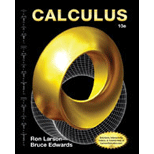Calculus
10th Edition
ISBN: 9781285057095
Author: Ron Larson, Bruce H. Edwards
Publisher: Cengage Learning#### Concept explainers#### Videos

Textbook QuestionChapter 8.1, Problem 81E

Comparing Antiderivatives

(a) Explain why the antiderivative y 1 = e x + C is equivalent to the antiderivative y 2 = C e x .

(b) Explain why the antiderivative y 1 = sec 2 x + C 1 , is equivalent to die antiderivative y 2 = tan 2 x + C .Students have asked these similar questions
VARIATION OF PARAMETERS  1. (D^2+1)y= sec tanx 2. (D^2-3D+2)y= 1/ 1+e^-x
Using Integration Techniques solve for the implicit solution. (xycos(x) + 1)dx + (xsin(x) + cos(x) + y^4)dy = 0
EQUATIONS REDUCIBLE TO EXACT EQUATIONS RULE 1 dx/dy = (x/y)2+(x/y)